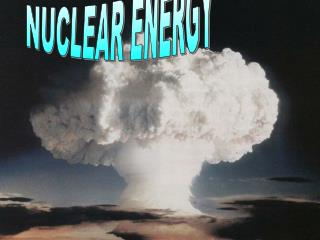DownloadDownload PresentationNUCLEAR ENERGY

# NUCLEAR ENERGY

Télécharger la présentation## NUCLEAR ENERGY

- - - - - - - - - - - - - - - - - - - - - - - - - - - E N D - - - - - - - - - - - - - - - - - - - - - - - - - - -
##### Presentation Transcript

1. NUCLEAR ENERGY NUCLEAR ENERGY

2. MassDefect Careful measurements show that the mass of a particular atom is always slightly less than the sum of the masses of the individual protons, neutrons and electrons of which the atom consists. e.g. a helium nucleus consists of 2 protons and 2 neutrons The difference between the mass of the atom and the sum of the masses of its parts is called  the  mass defect  (Dm). 2 protons & 2 neutrons Helium atom

3. 1 amu = 1/12 of the mass of an atom of Carbon-12 1 atomic mass unit ( amu ) = 1.661 x 10-27 kg mn = mass of a neutron (1.008665 amu) mp = mass of a proton   (1.007277 amu) me = mass of an electron   (0.000548597 amu) Z = PROTON NUMBER(ATOMIC NUMBER) AND ALSO EQUALS THE NUMBER OF ELECTRONS A = NUCLEON NUMBER(MASS NUMBER) AND ALSO EQUALS THE NUMBER OF PROTONS + NEUTRONS THE NUMBER OF NEUTRONS MUST BE A - Z Dm = [ Z(mp + me) + (A-Z)mn ] - matom

4. For Helium the mass defect will be Dm = [ 2(1.007277 + 0.000548597 ) + 2 x 1.008665 ] – 4.021435 = 0. 0115462 amu 0. 0115462 amu x 1.661 x 10-27 = 1. 91782 x 10-29 kg Using Einstein’s E = mc2,this is equivalent to a loss of energy given by E = 1.91782 x 10-29 x (3 x 108)2 Joules = 1.726 x 10-12 J This figure is the BINDING ENERGY of the Helium nucleus. THE BINDING ENERGY of a nucleus is defined as the energy which must be input to separate all of its protons and neutrons.

5. Binding Energies are usually expressed in MeV 1 amu = 931.3 MeV The binding energy of the Helium nucleus is therefore 0. 0115462 amu x 931.3 = 10.75 MeV To compare the stabilities of different nuclei, Binding Energies PER NUCLEON in the nucleus are compared. For Helium this will be 10.75 ÷ 4 = 2.69 MeV per nucleon The higher the binding energy per nucleon, the greater the stability of the nucleus

6. 56Fe 8.8 MeV 238U BINDING ENERGY Per NUCLEON 2H NUCLEON NUMBER Iron is the most stable nucleus

7. 0 250 A BE/A BE/A 9.0 A ALTERNATIVELY As energy is lost when nuclei are synthesised

8. IRON FISSION FUSION Heavy nuclei may increase their stability by Nuclear Fission Light nuclei may increase their stability by Nuclear Fusion

9. Belt of Stable Isotopes Neutron number, N = A - Z Proton number, Z Belt of Stability As atomic mass increases, the neutron to proton ratio for stable nuclei increases because proton-proton repulsion becomes significant, as the number of protons increases. Cohesive nuclear forces arise form neutrons, so the neutron to proton ratio must increase for heavier elements. N = Z For helium He- 4 the N:P ratio is 1 : 1 For uranium U- 238 the ratio is 1 : 1.6

10. Nuclear Fissionis the fragmentation of heavy nuclei to form lighter, more stable ones. The Fission of U - 235 This is only one of several fissures that are possible. On average 2.5 neutrons are released

11. Neutrons released in the fission of 235U can induce three more fissions, then nine, and so on leading to a chain reaction.

12. Critical massis the mass required for the chain reaction to become self-sustaining. neutron lost Cause more fission Get lost Be absorbed by an atom Some neutrons may : For a chain reaction to be self sustaining, every fission must produce at least one more neutron that will initiate further fissions.

13. Nuclear Reactor Boron control rods absorb neutrons to control the reaction Pressure vessel and biological shield To heat exchanger where steam is produced Fuel Rods remain in reactor until spent Graphite Moderator slows neutrons to thermal energies [1 MeV] Coolant [CO2 or H2O] to extract energy